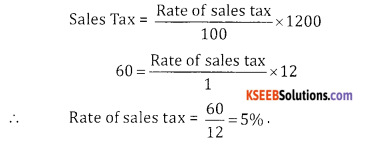# 2nd PUC Basic Maths Question Bank Chapter 12 Sales Tax and Value Added Tax Ex 12.1

Students can Download Basic Maths Exercise 12.1 Questions and Answers, Notes Pdf, 2nd PUC Basic Maths Question Bank with Answers helps you to revise the complete Karnataka State Board Syllabus and score more marks in your examinations.

## Karnataka 2nd PUC Basic Maths Question Bank Chapter 12 Sales Tax and Value Added Tax Ex 12.1

Part – A

2nd PUC Basic Maths Sales Tax and Value Added Tax Ex 12.1 Two or Three Marks Questions with Answers

Question 1.
Abhishek purchase a bicycle costing ₹ 12,000. If the rate of sales tax is 9%. Calculate the total amount payable by him.
Solution:
Given C.P = ₹ 12,000, Sales Tax = 9%
9 Sales Tax amount = $$\frac{9}{100}$$ × 12000 = 1080
∴ Amount payable by time = 12,000 + 1080 = 13,080.

Question 2.
Ramu paid ₹ 60 as sales tax on a Titan Raga watch worth ₹ 1200. Find the rate of sales tax.
Solution:
Given C.P = 1,200, Sales Tax = ₹ 60, rate = ?Question 3.
Refrigerator is marked for sales for ₹ 17,000 which include sales tax at 10% Calculate the sales tax in .
Solution:
Given S.P = ₹ 17,000, Sales Tax = 10% Let
C.P = x₹
W.K.T selling price = CP + Sales Tax
17,000 = x + 10% ofQuestion 4.
Bharath bought a shirt for ₹ 336, including 12% sales tax and a necktie for ₹ 110 including 10% sales tax. Find the printed price of shirt and necktie together,
Solution:
Given S.P = ₹ 336, S.P (neck tie) = 110/
Let C.P of shirt = x, & C.P of neck tie = y
Here, setting price of shirt = C.P + 12% of C.P
336 = x + $$\frac{12 x}{100}$$
336 = $$=\frac{112 x}{100}$$ ⇒ x = $$\frac{33600}{112}$$
Selling price of neck tie = C.P + 10% of C.P
110 = y + $$\frac{10 y}{100}$$
110 = $$\frac{10 y}{100}$$ ⇒ $$\frac{1100}{11}$$ = 100
∴ Printed price of shirt & neck tie = 300 + 100 = ₹ 400Question 5.
A furniture dealers sold furniture for ₹ 21,000 and added 5% S.T. to the quoted price. The pay for the same item if the ST is reduced to 6%.
Solution:
Given S.P = 21,000, Sales tax = 5%, Discount = ?
S.P = C.P + 5% of S.T on C.P
21,000 = x+ $$\frac{5 x}{100}$$
21000 = $$\frac{105 x}{100}$$
x = $$\frac{21000 \times 100}{105}$$ = 20000
∴ Tax paid = 1000
Let the discount be y%
∴ $$\frac{20000}{100} y$$ = 1000
y = $$\frac{1000}{200}$$ = 5%

Question 6.
A shopkeeper sells an item at the price of ₹ 810 including ST of 8% what should a customer pay for the same item if the ST is reduced to 6%.
Solution:
Given S.P = 810, S.T = 8%
S.P = C.P + 8% of C.P
810 = $$x+\frac{8 x}{100}=\frac{108 x}{100}$$
⇒ x = $$\frac{81000}{108}$$ = 750
∴ Cost price 750
S.P=750 + $$\frac{750 \times 6}{100}$$ = 750+ 45 = 795
Customer pay ₹ 795 for the same item if the S.T is reduced to 6%.Question 7.
The price of T.V. set inclusive of sales tax of 9% is ₹ 13.407. Find its marked price. If the S.T. is increased to
13% how much more does the customer Sneha pay for the T.V.
Solution:
Given S.T = 9%,
S.P = 13,407
S.P = C.P + 9% of C.P
9x 13,407 = x + $$\frac{9 x}{100}$$
13407 =$$\frac{109 x}{100}$$ ⇒ x = $$\frac{13407 \times 100}{109}$$ = 12300
Sales tax is at 13% i.e., 13% -9% = 4%
Sales tax = 12300 x $$\frac{4}{100}$$ = ₹ 492
∴ Customer should pay 492 more.

error: Content is protected !!